Recognized as:
Baylor University (326-41-438)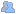Greater Western Library Alliance GWLA 2007 3707 (621-34-740)
658908 Greater Western Library Alliance Kluwer (merge with GWLA 2006 con acct when possible) (816-71-771)
Welcome!
Saved Items
 Journal Article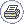Bilateral Laplace Transforms on Time Scales: Convergence, Convolution, and the Characterization of Stationary Stochastic Time Series
 (1) Department of Mathematics, Baylor University, One Bear Place #97328, Waco, TX 76798-7328, USA
 (2) Department of Electrical and Computer Engineering, Baylor University, One Bear Place #97356, Waco, TX 76798-7356, USA

Received: 30 May 2009  Revised: 29 September 2009  Published online: 30 April 2010

Abstract
The convergence of Laplace transforms on time scales is generalized to the bilateral case. The bilateral Laplace transform of a signal on a time scale subsumes the continuous time bilateral Laplace transform, and the discrete time bilateral z-transform as special cases. As in the unilateral case, the regions of convergence (ROCs) time scale Laplace transforms are determined by the time scale’s graininess. ROCs for the bilateral Laplace transforms of double sided time scale exponentials are determined by two modified Hilger circles. The ROC is the intersection of points external to modified Hilger circle determined by behavior for positive time and the points internal to the second modified Hilger circle determined by negative time. Since graininess lies between zero and infinity, there can exist conservative ROCs applicable for all time scales. For continuous time (ℝ) bilateral transforms, the circle radii become infinite and results in the familiar ROC between two lines parallel to the imaginary z axis. Likewise, on ℤ, the ROC is an annulus. For signals on time scales bounded by double sided exponentials, the ROCs are at least that of the double sided exponential. The Laplace transform is used to define the box minus shift through which time scale convolution can be defined. Generalizations of familiar properties of signals on ℝ and ℤ include identification of the identity convolution operator, the derivative theorem, and characterizations of wide sense stationary stochastic processes for an arbitrary time scales including autocorrelation and power spectral density expressions.

Keywords  Time scales - Laplace transform -  z-transforms - Region of convergence - Hilger circle - Stationarity - Autocorrelation - Power spectral density - Hilger delta

This work was supported by National Science Foundation grant CMMI #0726996.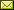John M. Davis (Corresponding author)Email: John_M_Davis@Baylor.eduIan A. GravagneEmail: Ian_Gravagne@Baylor.eduRobert J. Marks IIEmail: r.marks@ieee.org

## References

 1. M. Bohner, A. Peterson, Dynamic Equations on Time Scales: An Introduction with Applications (Birkhäuser, Boston, 2001)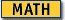2. C.J. Chyan, J.M. Davis, J. Henderson, W.K.C. Yin, Eigenvalue comparisons for differential equations on a measure chain. Electron. J. Differ. Equ. 1998, 35 (1998), 7 pp.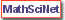3. J.M. Davis, J. Henderson, K.R. Prasad, Upper and lower bounds for the solution of the general matrix Riccati differential equation on a time scale. J. Comput. Appl. Math. 141, 133–145 (2002)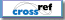4. J.M. Davis, I.A. Gravagne, B.J. Jackson, R.J. Marks II, A.A. Ramos, The Laplace transform on time scales revisited. J. Math. Anal. Appl. 332, 1291–1307 (2007)5. J.M. Davis, I.A. Gravagne, R.J. Marks II, Convergence of causal Laplace transforms on time scales. Circuits Syst. Signal Process (2010). doi:10.1007/s00034-010-9182-8 6. I.A. Gravagne, J.M. Davis, J. Dacunha, R.J. Marks II, Bandwidth sharing for controller area networks using adaptive sampling, in Proc. Int. Conf. Robotics and Automation (ICRA), New Orleans, LA, April 2004, pp. 5250–5255 7. S. Hilger, Ein Masskettenkalkül mit Anwendung auf Zentrumsmannigfaltigkeiten. Ph.D. thesis, Universität Würzburg (1988) 8. R.J. Marks II, Introduction to Shannon Sampling and Interpolation Theory (Springer, New York, 1991)9. R.J. Marks II (ed.), Advanced Topics in Shannon Sampling and Interpolation Theory (Springer, Berlin, 1993) 10. R.J. Marks II, Handbook of Fourier Analysis and Its Applications (Oxford University Press, London, 2009)11. R.J. Marks II, I. Gravagne, J.M. Davis, J.J. DaCunha, Nonregressivity in switched linear circuits and mechanical systems. Math. Comput. Model. 43, 1383–1392 (2006)12. R.J. Marks II, I.A. Gravagne, J.M. Davis, A generalized Fourier transform and convolution on time scales. J. Math. Anal. Appl. 340(2), 901–919 (2008)13. A. Papoulis, Probability, Random Variables and Stochastic Processes (McGraw-Hill, New York, 1968) 14. A. Papoulis, Generalized sampling expansion. IEEE Trans. Circuits Syst. CAS-24, 652–654 (1977)15. A. Papoulis, Signal Analysis (McGraw-Hill, New York, 1977)16. K. Yao, J.B. Thomas, On some stability and interpolating properties of nonuniform sampling expansions. IEEE Trans. Circuit Theory CT-14, 404–408 (1967) 17. K. Yao, J.B. Thomas, On a class of nonuniform sampling representation, in Symp. Signal Transactions Processing (Columbia University Press, New York, 1968)
Find
 Within all content Within this journal